# 1、OpenCV to detect how missing tooth in equipment

Hello everyone. I am just starting with OpenCV and still a bit lost.

Is OpenCV helpful to detect the position of a missing object (tooth for example)?

I would like to build a code to analyze an image of an equipment and detect which tooth is missing and its position.

For example in the image attached below of an equipment that has 9 teeth normally: the code should show a message that the 2nd tooth is missing.

Is this possible with OpenCV? If yes, where do I start?

Attached you will find the figure.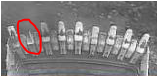## convexityDefects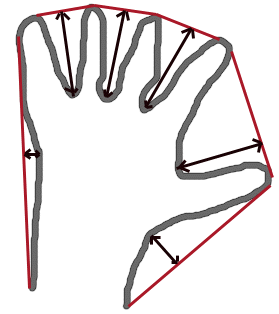# 2、Detect equipment in desired position

Hello everyone!

Is It possible to evaluate some pictures and save them Just when the object is in some desired position?

For example: monitoring a vídeo of an equipment that moves in and out of the scene. I would like to detect the time when the equipment is fully in the scene and then save It in a desired folder.

Thanks a Lot and have a great weekend

# 3、Does findContours create duplicates

Hi,I'm considering a binary image from which I extract its edges using cv::Canny. Consequently, I perform cv::findContours, storing all the contours points coordinates in a

vector < vector < Point > >

I noticed that the number of pixels (Points) in this structure is greater than the number of pixels I get by computing

vector<point> white_pixels;
findNonZero(silhouette, white_pixels);

on the same image.

Therefore I'm wondering if this happens because findContours includes duplicate points in its result or because findNonZero is less precise.

E.g. on a 200x200 sample image with the first method I get 1552 points while with the second I get 877.

In case the first hypothesis is correct, is there a way to either ignore the duplicates or remove them?

a、

In case of a Canny edge image findContours will find most of the contour points twice, because the line width is only 1 and findContours is looking for closed contours.

To remove duplicates you can map the contour points to an empty (zero) image.For every contour point add 1 at the image position and only copy the contour point to your new contour vector if the value at this position is zero. That would be the easiest way I think.

Thanks! Actually, I did what you suggested using the same sample image and also counting how many pixels were set to 1 (without repetitions). I ended up with having exactly 877 white pixels in the new Mat::zeros image. At this point, i think the result I get by using "findNonZero" is correct, accurate and in this case more efficient since I can avoid the double for loop I used for mapping the contour points for this test.

b、My first answer was wrong. @matman answer is good but I think problem is in blurred image.

If you don't blur enough image you will have many double points. You shape become a line but at beginning your shape could be a rectangle.

if you find double point in your contour may be you have to increase blurring (shape like 8 could be a problem). For fractal shape (or with a large rugosity) it is difficult to delete those points.

In this example I use :surface rectangle 6 (width 3 and height 2)

canny witout blurring Image 0

canny with blur size 3 image 1

Canny with blur size 5 image 2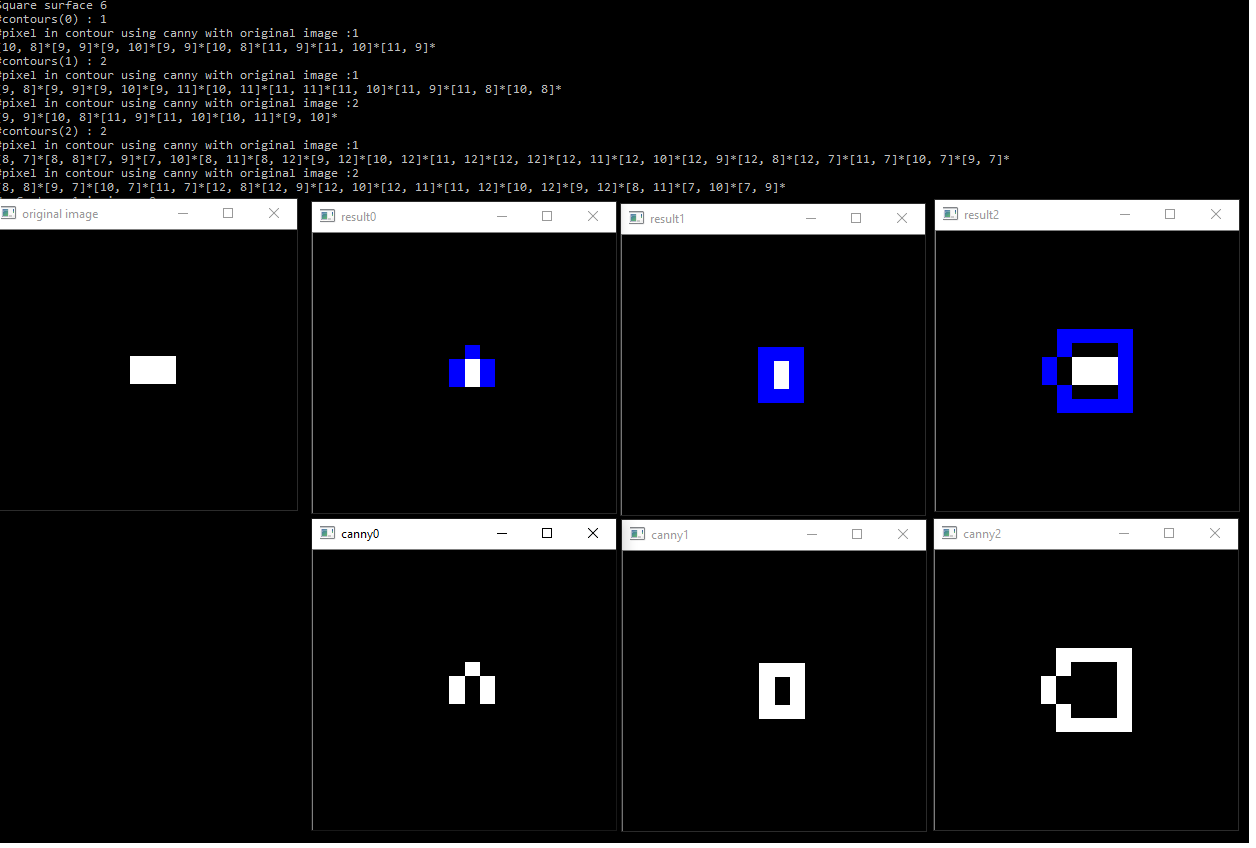Source file

{    Mat x = Mat::zeros(20,20,CV_8UC1);    Mat result = Mat::zeros(20,20,CV_8UC3);    vector<Vec3b> c = { Vec3b(255, 0, 0), Vec3b(0,255,0),Vec3b(0,0,255),Vec3b(255, 255, 0),Vec3b(255, 0, 255),Vec3b(0, 255, 255) };    for (int i=9;i<=10;i++)        for (int j = 9; j <= 11; j++)        {        x.at<uchar>(i,j)=255;        result.at<Vec3b>(i,j)=Vec3b(255,255,255);        }    imwrite("square.png",x);    Mat idx;    findNonZero(x,idx);    cout << "Square surface " << idx.rows<<endl;    vector<vector<vector<Point> >> contours(3);    vector<Vec4i> hierarchy;    double thresh=1;    int aperture_size=3;    vector<Mat> xx(3);    vector<Mat> dst(3);    for (size_t i = 0; i < xx.size(); i++)    {        if (i==0)            xx[i]=x.clone();        else            blur(x, xx[i], Size(static_cast<int>(2*i+1),static_cast<int>(2*i+1)));        Canny(xx[i], dst[i],thresh, thresh, 3,true );        namedWindow(format("canny%d",i),WINDOW_NORMAL);        namedWindow(format("result%d",i),WINDOW_NORMAL);        imshow(format("canny%d",i),dst[i]);        findContours(dst[i],contours[i], hierarchy, CV_RETR_TREE, CV_CHAIN_APPROX_NONE, Point(0, 0));   }     namedWindow("original image",WINDOW_NORMAL);    imshow("original image",x);   /*        Mat dx,dy,g;    Sobel(x, dx, CV_16S, 1, 0, aperture_size, 1, 0, BORDER_REPLICATE);    Sobel(x, dy, CV_16S, 0, 1, aperture_size, 1, 0, BORDER_REPLICATE);    namedWindow("gradient modulus",WINDOW_NORMAL);    g = dx.mul(dx) + dy.mul(dy);    imshow("gradient modulus",g);    findContours(x,contours, hierarchy, CV_RETR_TREE, CV_CHAIN_APPROX_NONE, Point(0, 0));    cout << "#contours : " << contours.size()<<endl;    if (contours.size() > 0)    {        for (size_t i = 0; i < contours.size(); i++)        {            cout << "#pixel in contour of original image :"<<contours[i].size()<<endl;            for (size_t j=0;j<contours[i].size();j++)                cout << contours[i][j] << "*";            cout<<endl;            drawContours(result, contours, i, c[i]);        }    }*/     size_t maxContour=0;    for (size_t k = 0; k < 3; k++)    {        cout << "#contours("<<k<<") : " << contours[k].size()<<endl;;        if (maxContour<contours[k].size())            maxContour= contours[k].size();        if (contours[k].size() > 0)        {            for (size_t i = 0; i<contours[k].size();i++)            {                cout << "#pixel in contour using canny with original image :"<<contours[i].size()<<endl;                for (size_t j=0;j<contours[k][i].size();j++)                    cout << contours[k][i][j] << "*";                cout<<endl;            }         }        else            cout << "No contour found "<<endl;     }    int index=0;    while (true)    {        char key = (char)waitKey();        if( key == 27 )            break;         if (key == '+')        {            index = (index+1)%maxContour;        }        if (key == '-')        {            index = (index-1);            if (index<0)                index = maxContour-1;        }        vector<Mat> result(contours.size());        for (size_t k = 0; k < contours.size(); k++)        {           result[k] = Mat::zeros(20,20,CV_8UC3);           for (int ii=9;ii<=10;ii++)                for (int jj = 9; jj <= 11; jj++)                {                    result[k].at<Vec3b>(ii,jj)=Vec3b(255,255,255);                }             if (index<contours[k].size())                drawContours(result[k], contours[k], static_cast<int>(index), c[index]);            else                cout << "No Contour "<<index<<" in image "<<k<<endl;            imshow(format("result%d",k),result[k]);        }        cout << "Contour "<<index<<endl;    }    exit(0);}

但是对于今天的我来说，分析重复轮廓的意义在哪里了？很多时候，我都只是找最大的外围轮廓就可以的。

# 4、FindContours Duplicate Points

I am using OpenCV 3.4.1 with VS2015 C++ on a Win10 platform.

My question relates to findContours and whether that should be returning duplicate points within a contour.

For example, I have a test image like this: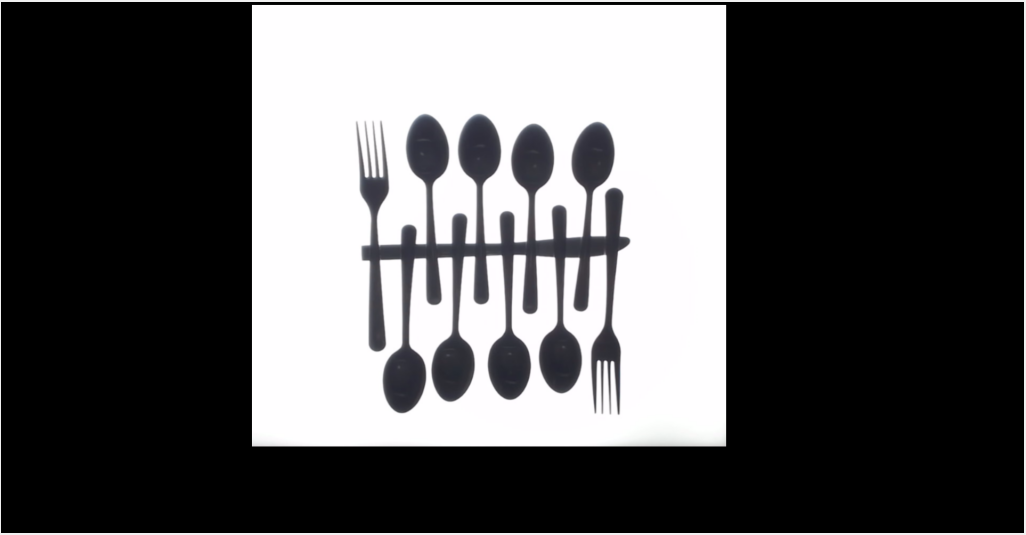I do Canny on it and then I run findContours like this:

findContours(this->MaskFrame,this->Contours,this->Hierarchy,CV_RETR_EXTERNAL,CV_CHAIN_APPROX_NONE);

When I check the resulting contours like this:

for (int x = 0; x < Images->Contours.size(); x++)for (int y = 0; y < Images->Contours[x].size(); y++)for (int z = y + 1; z < Images->Contours[x].size(); z++)if (Images->Contours[x][y] == Images->Contours[x][z])printf("Contours duplicate point: x: %d, y: %d z: %d\n", x, y, z);

I can see there there are many/hundreds of duplicate points within a given contour.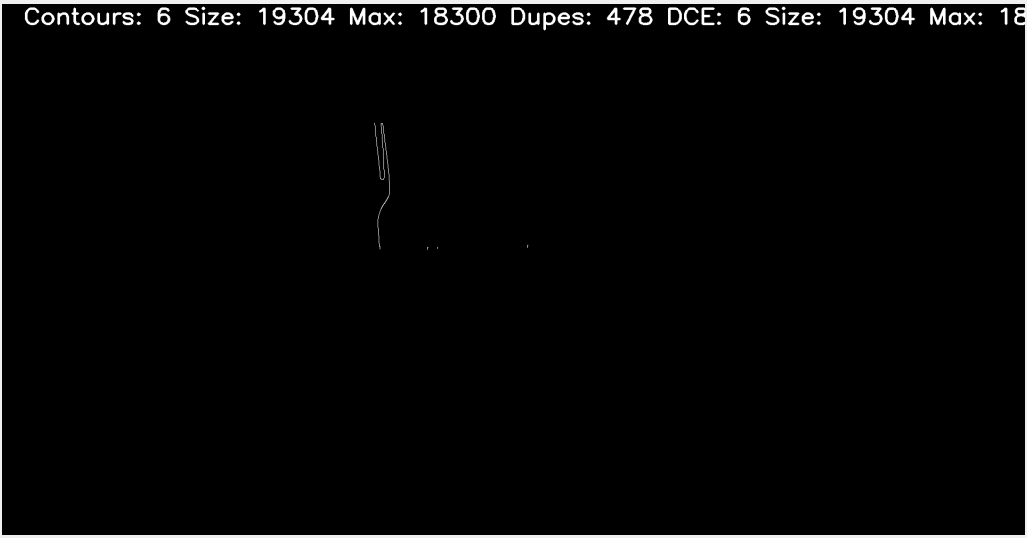The presence of the duplicates seems to cause a problem with the drawContours function.
Nevertheless, this image shows that 6 contours were detected with ~19,000 points comprising all the contours, the largest contour has ~18,000 points, but there are 478 points that are duplicated within a contour.

However, this only seems to occur if the total number of points in a given contour is fairly large, e.g., > 2000 points.If I arrange the image so that no contour has more than ~2000 points, as below, then there are no duplicates.In this image, there are 11 contours, there are ~10,000 points comprising all the contours, with the largest contour having ~1,600 points, and no duplicates.

Before I try and get deep into findContours or something else, I thought I would ask: anyone have any ideas why I am seeing duplicate points within a contour?

Thanks for any help.

# 5、how to find the REAL width and height of contours这道题目是我问的，而且也给出了最优回答，应该说是有价值的。

there is a image of 3 shapes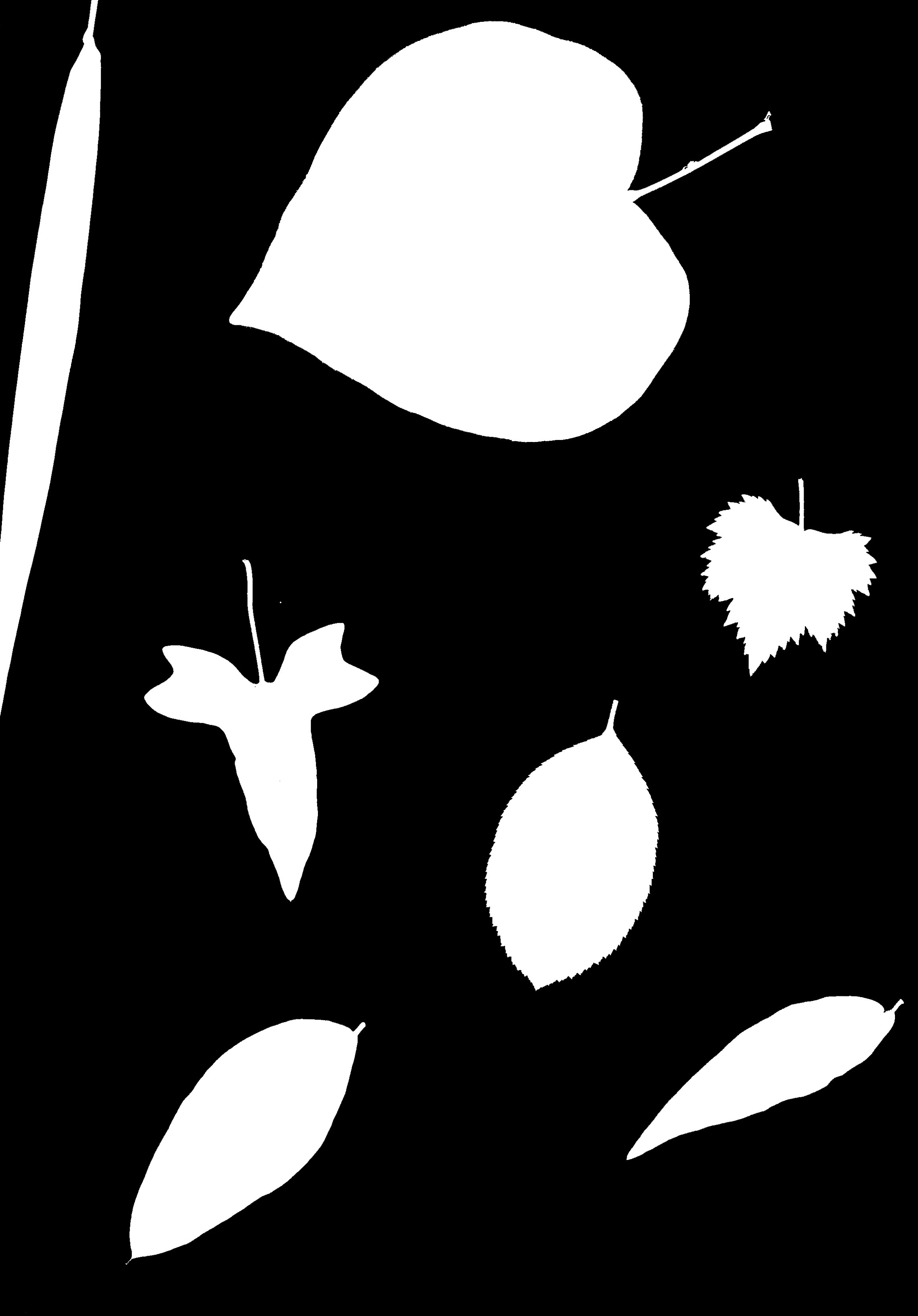int main( int argc, char** argv ){    //read the image    Mat img = imread("e:/sandbox/leaf.jpg");    Mat bw;    bool dRet;    //resize    pyrDown(img,img);    pyrDown(img,img);    cvtColor(img, bw, COLOR_BGR2GRAY);    //morphology operation      threshold(bw, bw, 150, 255, CV_THRESH_BINARY);    //bitwise_not(bw,bw);    //find and draw contours    vector<vector<Point> > contours;    vector<Vec4i> hierarchy;    findContours(bw, contours, hierarchy, CV_RETR_LIST, CV_CHAIN_APPROX_NONE);    for (int i = 0;i<contours.size();i++)    {        RotatedRect minRect = minAreaRect( Mat(contours[i]) );        Point2f rect_points;        minRect.points( rect_points );         for( int j = 0; j < 4; j++ )            line( img, rect_points[j], rect_points[(j+1)%4],Scalar(255,255,0),2);    }    imshow("img",img);    waitKey();    return 0;}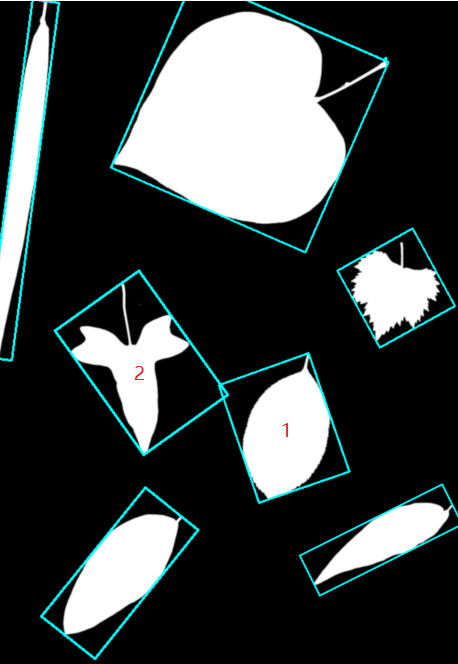but ,in fact ,the contour 1 and contour 2 which i fingure out in RED do not get the right widht and height.

what i want should be this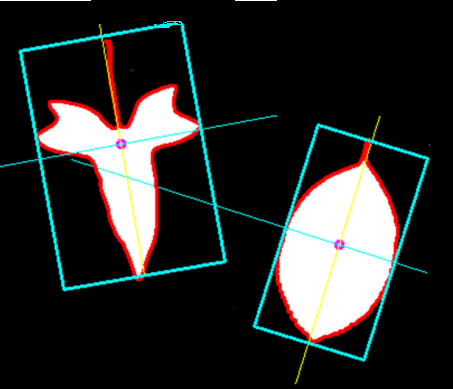i did't find the appropriate function or any Doc from Opencv to do this workIt's been bothering me for daysany help will be appreciate!

e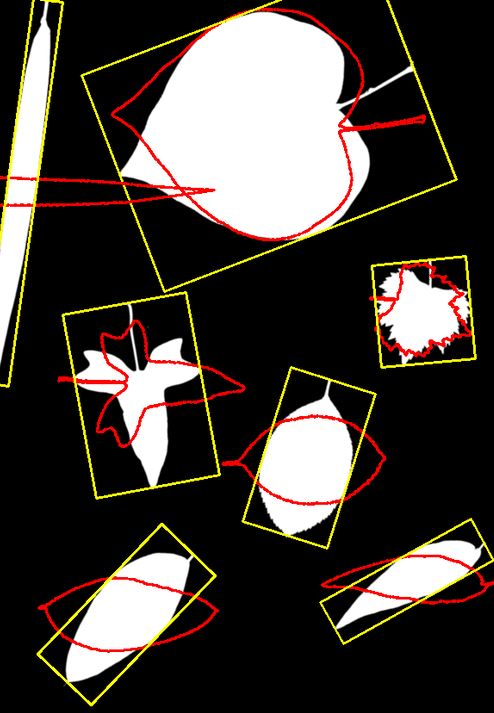#include "opencv2/imgcodecs.hpp"#include "opencv2/highgui.hpp"#include "opencv2/imgproc.hpp"#include "opencv2/photo.hpp"using namespace std;using namespace cv;#define DEBUG FALSEPoint2f GetPointAfterRotate(Point2f inputpoint,Point2f center,double angle){    Point2d preturn;    preturn.x = (inputpoint.x - center.x)*cos(-angle) - (inputpoint.y - center.y)*sin(-angle)+center.x;    preturn.y = (inputpoint.x - center.x)*sin(-angle) + (inputpoint.y - center.y)*cos(-angle)+center.y;    return preturn;}Point GetPointAfterRotate(Point inputpoint,Point center,double angle){    Point preturn;    preturn.x = (inputpoint.x - center.x)*cos(-1*angle) - (inputpoint.y - center.y)*sin(-1*angle)+center.x;    preturn.y = (inputpoint.x - center.x)*sin(-1*angle) + (inputpoint.y - center.y)*cos(-1*angle)+center.y;    return preturn;}double getOrientation(vector<Point> &pts, Point2f& pos,Mat& img){    //Construct a buffer used by the pca analysis    Mat data_pts = Mat(pts.size(), 2, CV_64FC1);    for (int i = 0; i < data_pts.rows; ++i)    {        data_pts.at<double>(i, 0) = pts[i].x;        data_pts.at<double>(i, 1) = pts[i].y;    }    //Perform PCA analysis    PCA pca_analysis(data_pts, Mat(), CV_PCA_DATA_AS_ROW);    //Store the position of the object    pos = Point2f(pca_analysis.mean.at<double>(0, 0),        pca_analysis.mean.at<double>(0, 1));    //Store the eigenvalues and eigenvectors    vector<Point2d> eigen_vecs(2);    vector<double> eigen_val(2);    for (int i = 0; i < 2; ++i)    {        eigen_vecs[i] = Point2d(pca_analysis.eigenvectors.at<double>(i, 0),            pca_analysis.eigenvectors.at<double>(i, 1));        eigen_val[i] = pca_analysis.eigenvalues.at<double>(i,0);    }    return atan2(eigen_vecs.y, eigen_vecs.x);}int main( int argc, char** argv ){    Mat img = imread("e:/sandbox/leaf.jpg");    pyrDown(img,img);    pyrDown(img,img);    Mat bw;    bool dRet;    cvtColor(img, bw, COLOR_BGR2GRAY);    threshold(bw, bw, 150, 255, CV_THRESH_BINARY);    vector<vector<Point> > contours;    vector<Vec4i> hierarchy;    findContours(bw, contours, hierarchy, CV_RETR_LIST, CV_CHAIN_APPROX_NONE);    for (size_t i = 0; i < contours.size(); ++i)    {        double area = contourArea(contours[i]);        if (area < 1e2 || 1e5 < area) continue;        Point2f* pos = new Point2f();        double dOrient =  getOrientation(contours[i], *pos,img);        int xmin = 99999;        int xmax = 0;        int ymin = 99999;        int ymax = 0;        for (size_t j = 0;j<contours[i].size();j++)        {            contours[i][j] = GetPointAfterRotate(contours[i][j],(Point)*pos,dOrient);            if (contours[i][j].x < xmin)                xmin = contours[i][j].x;            if (contours[i][j].x > xmax)                xmax = contours[i][j].x;            if (contours[i][j].y < ymin)                ymin = contours[i][j].y;            if (contours[i][j].y > ymax)                ymax = contours[i][j].y;        }        Point lt = Point(xmin,ymin);        Point ld = Point(xmin,ymax);        Point rd = Point(xmax,ymax);        Point rt = Point(xmax,ymin);            drawContours(img, contours, i, CV_RGB(255, 0, 0), 2, 8, hierarchy, 0);        lt = GetPointAfterRotate((Point)lt,(Point)*pos,-dOrient);        ld = GetPointAfterRotate((Point)ld,(Point)*pos,-dOrient);        rd = GetPointAfterRotate((Point)rd,(Point)*pos,-dOrient);        rt = GetPointAfterRotate((Point)rt,(Point)*pos,-dOrient);        line( img, lt, ld,Scalar(0,255,255),2);        line( img, lt, rt,Scalar(0,255,255),2);        line( img, rd, ld,Scalar(0,255,255),2);        line( img, rd, rt,Scalar(0,255,255),2);    }    return 0;}

# 6、Is findContours fast enough ?

For current vision algorithms (e.g. object detection, object enhancing) does findCountours perform fast enough ? I've studied the algorithm behind it  and by a first look it's rather difficult to perform in parallel especially on SIMD units like GPUs. I took a usage example from  and did a simple trace of findCountours on  and . While  requires 1ms,  requires about 40ms (AMD 5400K @3.6ghz). If high resolution video processing frame by frame is considered these results could be problematic. I think i may have an ideea for a faster algorithm oriented towards SIMD units. So i would like some feedback from people who have worked in vision problems to the question:

Is findCountours fast enough for current problems on current hardware ? Would improving it help in a significant way any specific algorithm ?

I am wondering how object detection and findContours work together. I am working fulltime on object detection using the viola-jones cascade classification, the latentSVM of felzenszwalb and the dollar channelftrs framework which are all state of the art techniques and none of them need findContours ...

It was more of an assumption than a confirmation. I previously worked on image vectorization (raster->vector, e.g. algorithm ,) and noticed that contour extraction was one of the problems on large scale images (such as maps). The question is actually more oriented towards "are there any problems that require fast contour extraction" or is there a scenario where "contour extraction is the slowest step" ? I think image vectorization is not enough for me to continue and invest time into this topic.

1

So you want insight in the fact 'is it interesting to improve the findContours algorithm' ?

1

Yes. I mean i have an idea how to make it faster but would like to write it for GPUs using OpenCL and that would take some time. And if i manage to improve it by a % margin why would anyone bother integrating it or checking it out if contour extraction itself isn't very used (assuming) hence the current OpenCV findCountour would be enough for most people.

1

It is usefull in many other cases. I for example use it to detect blobs after an optimized segmentation. Increasing the blob detection process is always usefull.

# 7、Circle detection,

I'm trying to find circles around the white disks (see example pictures below). The background is always darker than the foreground. The dots can sometimes be a bit damaged and therefore not of a full disk shape. Nevertheless I would like to fit circles as accurate as possible to the white disks. The found circles should all have a very similar radius (max. 5-10% difference). The circle detection should be focusing on determining the disks radius as accurate as possible.

What I tried so far: - Hough circle detection with various settings and preprocessing of the images - findContours

I was not able to produce satisfying results with these two algorithms. I guess it must have something to do how I preprocess the image but I have no idea where to start. Can anyone give me any advice for a setup that will solve this kind of problem?

Thank you very much in advance!Like @LBerger linked (but I do not really like links only responses) you should apply the following pipeline.

• Binarize your image, using for example OTSU thresholding
• Apply the distance transform to find the centers of all disk regions
• Use those to apply a watershed segmentation

It will give you a result, like described in the tutorial and should look something like this:# 8、Can I resize a contour?

I have an application that detects an object by finding its contour. Because the image where I search for the object may be at a very big scale is affecting my detection because the contour can be too long and I skip the contours longer than a threshold. For fixing this, I have thought of resizing the image larger than a maximum size to that maw size. Doing so, I am detecting the object in the smaller image, and when drawing the contour on the initial image, I am getting a "wrong detection". Is there a possibility to resize the contour?

Nice, it is working, and it is working very nice. More, your idea of resizing the mask is introducing errors, because the cv::fillPoly is introducing errors (small "stairs") and resizing it is just making the errors to appear in the new contour, and they are even bigger.

#include "opencv2/imgproc.hpp"#include "opencv2/highgui.hpp"using namespace cv;int main( int argc, char** argv ){    vector<vector<Point> > contours;    Mat img = Mat::zeros( 500, 500, CV_8UC1 );    circle( img, Point(250,250), 100, Scalar(255) );    findContours( img, contours, RETR_LIST, CHAIN_APPROX_SIMPLE);    fillConvexPoly( img, Mat( contours ) / 2, Scalar(255)); // draws contour resized 1x/2    polylines( img, Mat( contours ) * 2, true, Scalar(255)); // draws contour resized 2x    imshow("result",img);    waitKey();    return 0;}You can use the following method to resize the contour by keeping the contour center like the morphology operation.

 void contourOffset(const std::vector<cv::Point>& src, std::vector<cv::Point>& dst, const cv::Point& offset) {    dst.clear();    dst.resize(src.size());    for (int j = 0; j < src.size(); j++)        dst[j] = src[j] + offset;}void scaleContour(const std::vector<cv::Point>& src, std::vector<cv::Point>& dst, float scale){    cv::Rect rct = cv::boundingRect(src);    std::vector<cv::Point> dc_contour;    cv::Point rct_offset(-rct.tl().x, -rct.tl().y);    contourOffset(src, dc_contour, rct_offset);    std::vector<cv::Point> dc_contour_scale(dc_contour.size());    for (int i = 0; i < dc_contour.size(); i++)        dc_contour_scale[i] = dc_contour[i] * scale;    cv::Rect rct_scale = cv::boundingRect(dc_contour_scale);    cv::Point offset((rct.width - rct_scale.width) / 2, (rct.height - rct_scale.height) / 2);    offset -= rct_offset;    dst.clear();    dst.resize(dc_contour_scale.size());    for (int i = 0; i < dc_contour_scale.size(); i++)        dst[i] = dc_contour_scale[i] + offset;    }void scaleContours(const std::vector<std::vector<cv::Point>>& src, std::vector<std::vector<cv::Point>>& dst, float scale){    dst.clear();    dst.resize(src.size());    for (int i = 0; i < src.size(); i++)        scaleContour(src[i], dst[i], scale);}        void main(){            std::vector<std::vector<cv::Point>> src,dst;            scaleContours(src,dst,0.95);         }

In the sample below, the green contour is main contour and the red contour is scaled contour with a coefficient of 0.95.posted @ 2018-09-01 08:57  jsxyhelu  阅读(507)  评论(0编辑  收藏  举报